# Freiheitssatz

(diff) ← Older revision | Latest revision (diff) | Newer revision → (diff)

independence theorem

A theorem originally proposed by M. Dehn in a geometrical setting and originally proven by W. Magnus [a1]. This theorem is the cornerstone of one-relator group theory.

The Freiheitssatz says the following: Let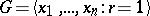be a group defined by a single cyclically reduced relator. If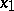appears in, then the subgroup ofgenerated by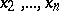is a free group, freely generated by.

In coarser language, the theorem says that ifis as above, then the only relations inare the trivial ones.

The Freiheitssatz can be considered as a non-commutative analogue of certain more transparent results in commutative algebra. For example, suppose that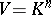is a linear space over a fieldwith a basis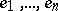. If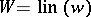is the subspace ofgenerated by a vector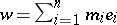with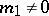, then the elements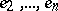are linearly independent modulo.

Magnus' method of proof of the Freiheitssatz relies on amalgamations (cf. also Amalgam; Amalgam of groups). This method initiated the use of these products in the study of infinite discrete groups.

One of the by-products of Magnus' proof was an extraordinary description of the structure of these groups, which allowed him to deduce that one-relator groups have solvable word problem (cf. also Identity problem; [a2]).

There are two general approaches to extending the Freiheitssatz. The first is concerned with the notion of the one-relator productof a familyof groups, where the elementis cyclically reduced and of syllable length at leastand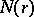is its normal closure in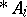. Some authors (see [a3]) give conditions for the factorsto inject into.

The second approach is concerned with multi-relator versions of the Freiheitssatz (see [a3] for a list of references). For example, the following strong result by N.S. Romanovskii [a4] holds: Lethave deficiency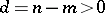. Then there exist a subset ofof the given generators which freely generates a subgroup of.